# Regular Polygon

Have you ever noticed the fascinating structure of the honeycomb? How does it look so perfect?It's because of the amazing six-sided identical shapes called hexagons, that connect to each other so perfectly! That's the beauty of nature!

In this mini-lesson, let us learn more about such shapes which are known as Regular Polygons along with regular polygon examples, not regular polygons, irregular polygons, and area of a regular polygon. Check out the interactive simulations to know more about the lesson and try your hand at solving a few interesting practice questions at the end of the page.

## Lesson Plan

 1 Definition of a Regular Polygon 2 Solved Examples 3 Challenging Questions! 4 Interactive Questions 5 Important Notes

## Definition of a Regular Polygon

According to the Euclidean Geometry, a polygon which is equiangular and equilateral is called a regular polygon.

In simple words, a regular polygon has all angles of the same measure and all sides of the same length.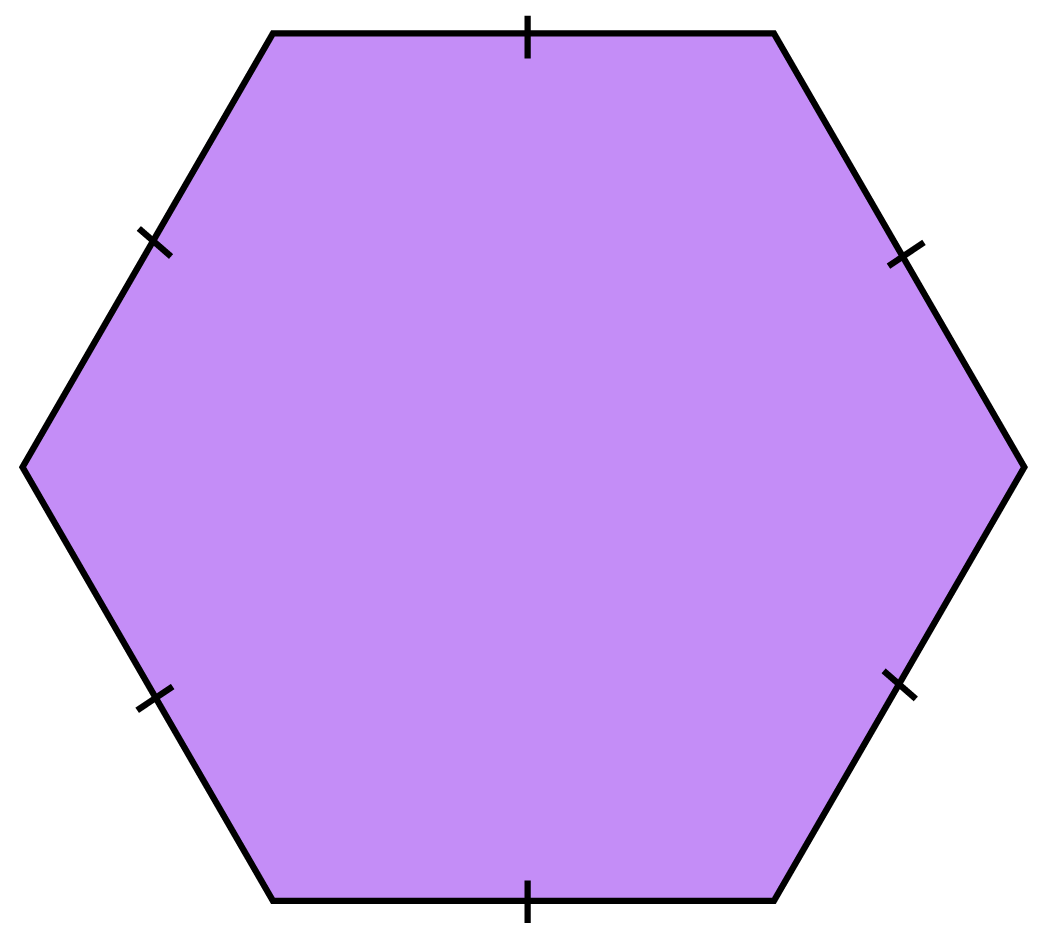## Properties of Regular Polygons

A polygon is considered to be a regular polygon if it fulfills the following properties:

• All its sides are equal
• All its interior angles are equal
• All its exterior angles are equal

Let us understand this with the example of an equilateral triangle ABC.

We will check whether an equilateral triangle fulfills all the three properties of a regular polygon or not.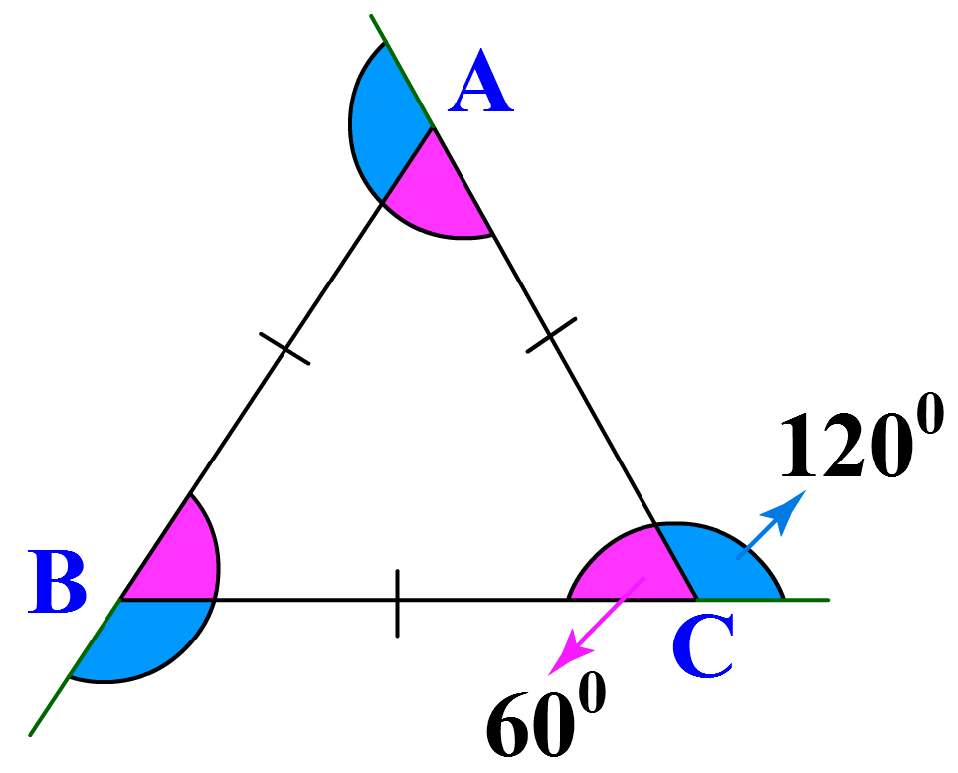We observe that:

Sides Interior Angles AB = BC = AC It fulfills the first property All the three interior angles are 60° (equal) It fulfills the second property All the exterior angles are 120° (equal) It fulfills the third property

Hence, triangle ABC is a regular polygon.

Let us take another example of a rectangle PQRS.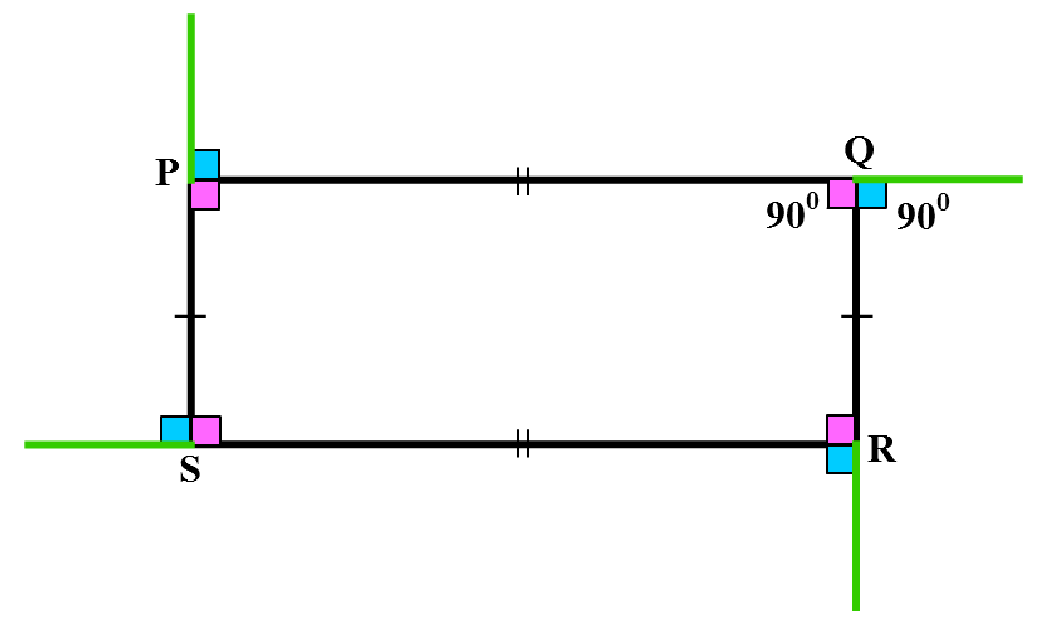We observe that:

Sides Interior Angles PQ = SR and PS = QR (All the sides are not equal, only the opposite sides are equal) So it does not fulfill the first property All the interior angles are 90° (equal) It fulfills the second property All the exterior angles are 90° (equal) It fulfills the third property

Hence, rectangle PQRS is not a regular polygon because it does not fulfill one property of a regular polygon.

## Irregular Polygons

A polygon whose sides are not of the same length and angles are not of the same measure are called Irregular Polygons.

In simple words, polygons which do not fulfill the properties of a regular polygon are not regular polygons, and hence, are known called irregular polygons.

For example: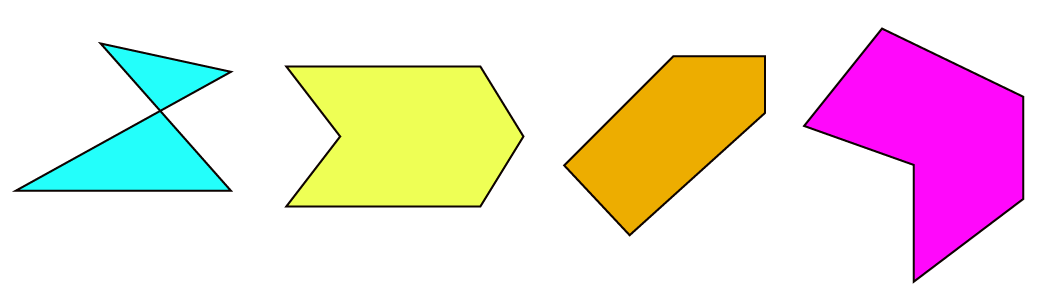Can you think of some irregular polygons around you?

## Parts of Regular Polygons

There are five basic parts of a regular polygon:

• Vertices
• Sides
• Interior Angles
• Exterior Angles
• Diagonals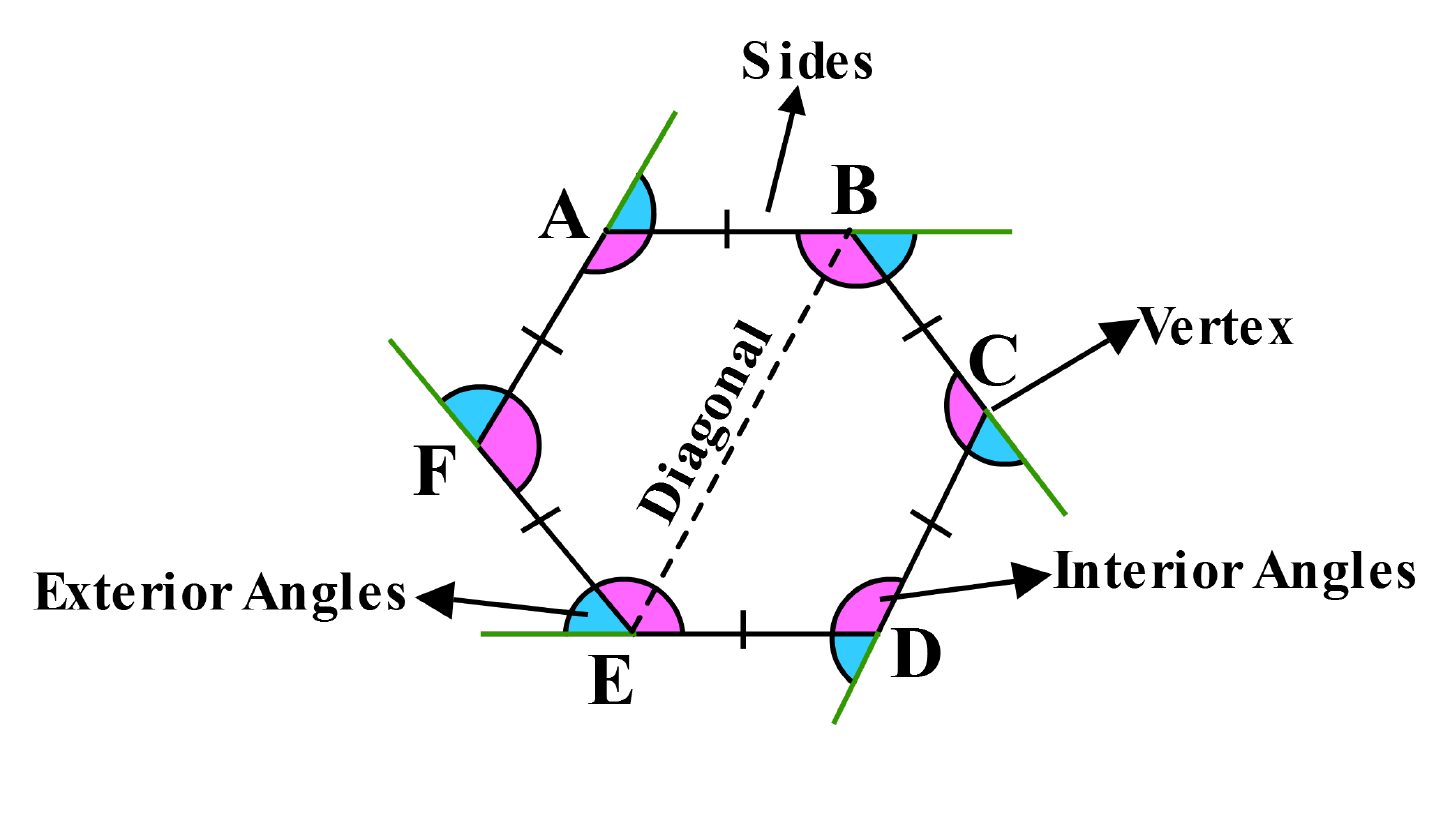Let us understand these with the example of a regular hexagon.

This regular hexagon has:

### Vertices

Vertices or corners A, B, C, D, E, F

### Sides

Equal sides: AB, BC, CD, DE, EF, FA

The number of sides of a regular polygon are denoted by the letter 'n' in formulas.

In this case, since the hexagon has 6 sides, n = 6.

### Interior Angles

The interior angles are formed between the adjacent sides inside the polygon and are equal to each other.

The number of interior angles are equal to the number of sides. This hexagon has 6 interior angles.

The value of an interior angle of a regular polygon can be calculated if the number of sides of the regular polygon are known.

### Exterior Angles

Each exterior angle of a regular polygon is formed by extending one side of the polygon and then the angle between that extension and the adjacent side is measured.

An exterior angle can be calculated if the number of sides of a regular polygon are known.

Each exterior angle of a regular polygon is equal and the sum of the exterior angles of a regular polygon is 360°.

### Diagonals

When any non-adjacent vertices inside a polygon are connected by a line segment, they form diagonals. In this hexagon, the number of diagonals that can be formed are nine.

Try this interactive simulation to check the interior angles of regular polygons.

Click on the names of the polygons to know the measure of each of their interior angles.

## Names of Regular Polygons

Observe the Polygons with their angles:

Number of Sides Name Interior Angles Exterior Angles
3

Equilateral Triangle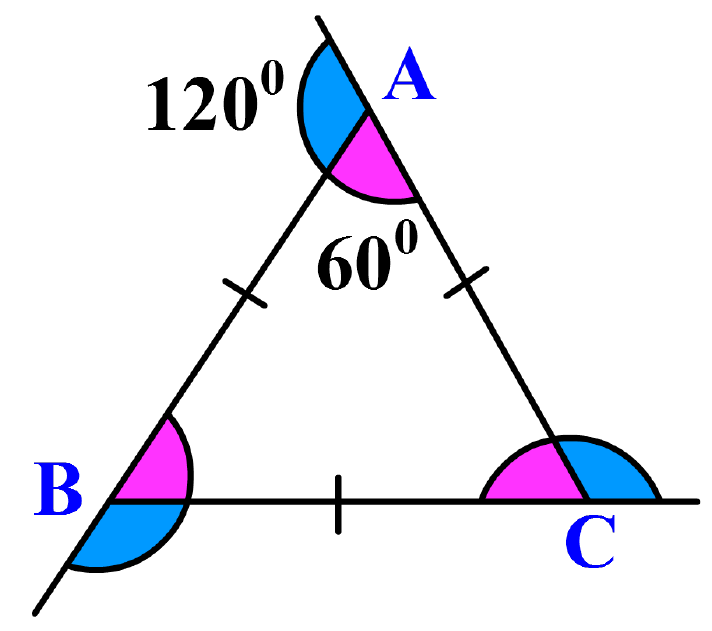3 angles of 60° 3 angles of 120°
4

Square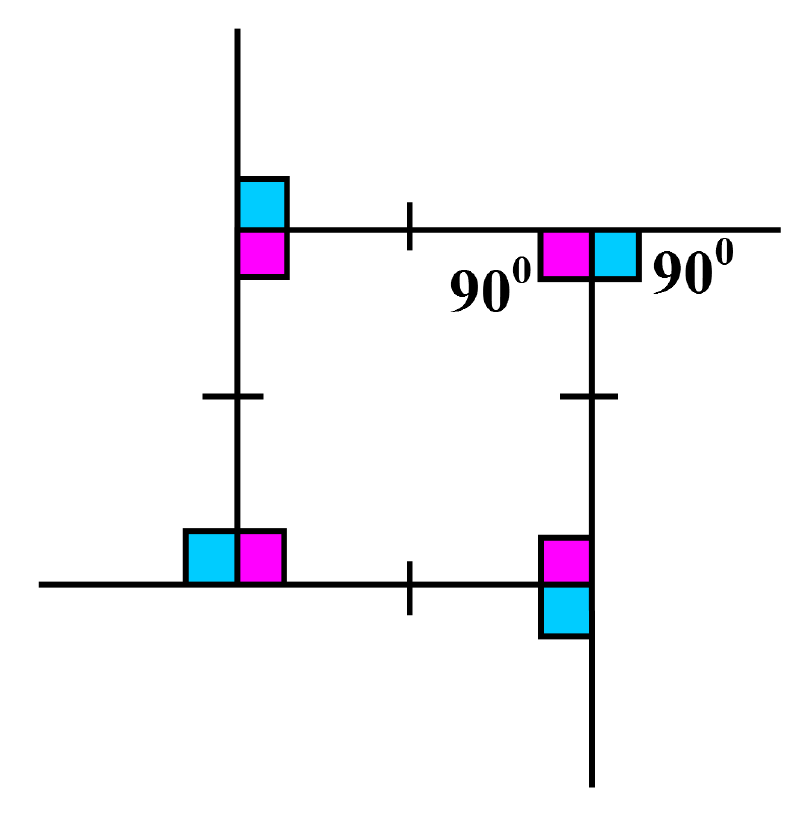4 angles of 90° 4 angles of 90°
5

Regular Pentagon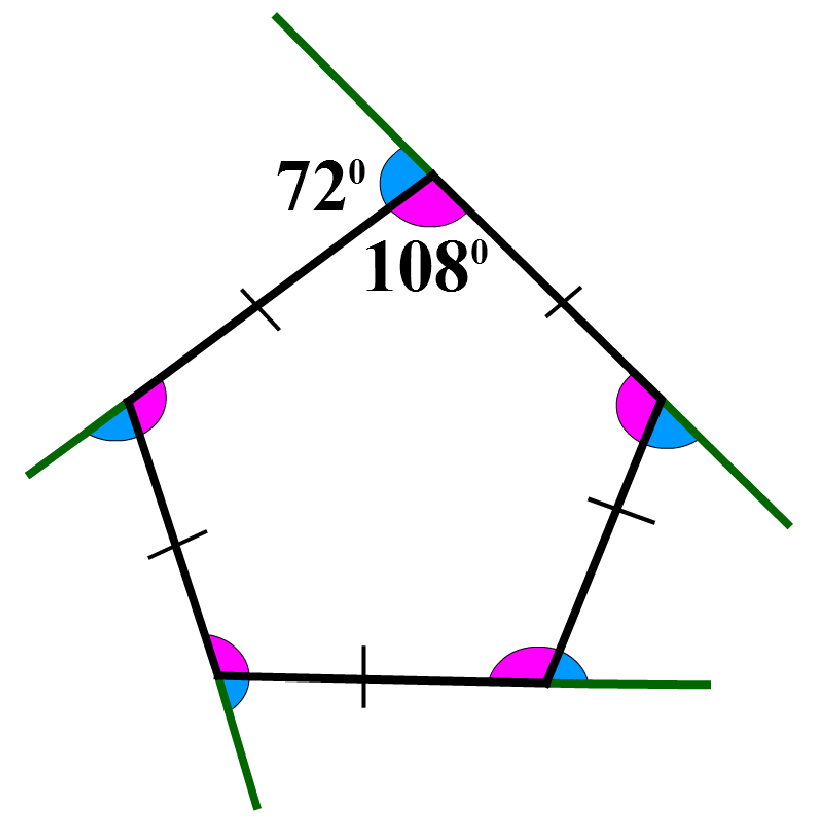5 angles of 108° 5 angles of 72°
6

Regular Hexagon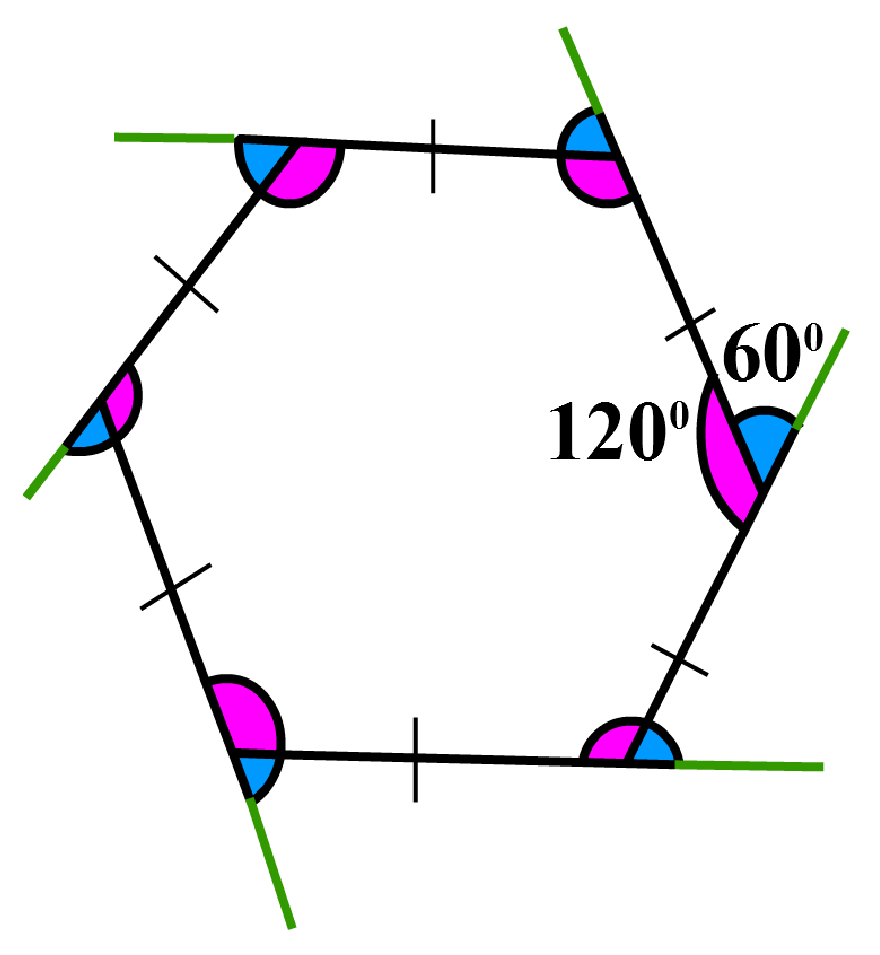6 angles of 120° 6 angles of 60°
7

Regular Heptagon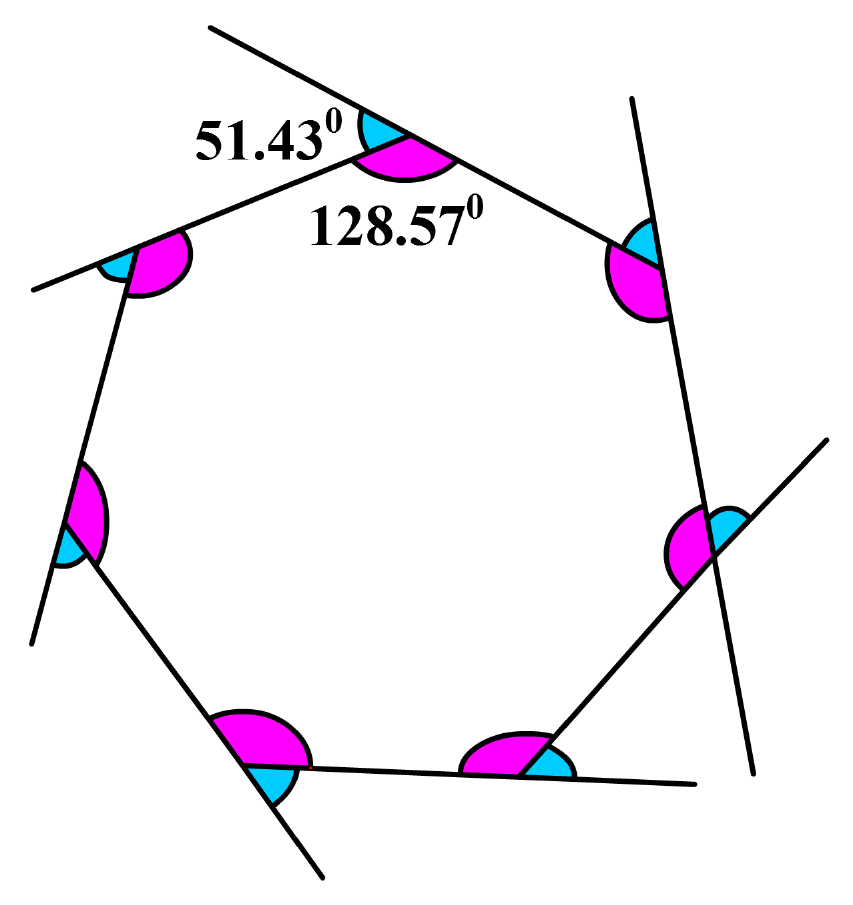7 angles of 128.57° 7 angles of 51.43°
8

Regular Octagon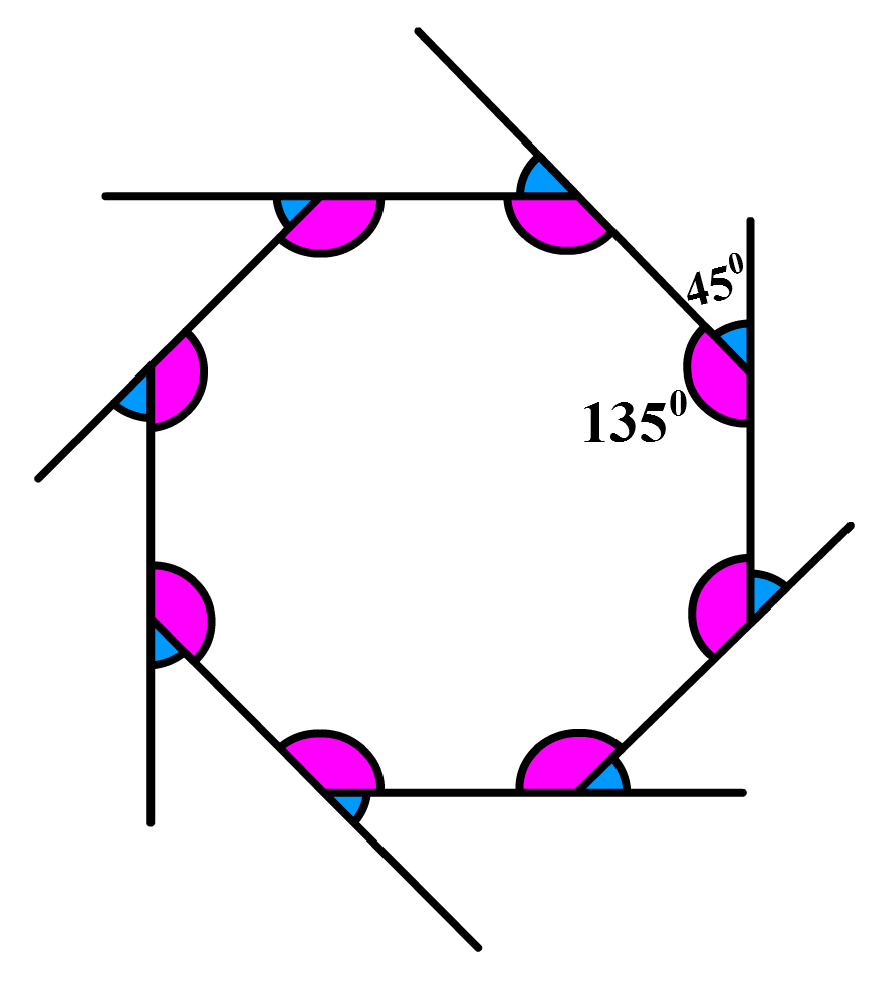8 angles of 135° 8 angles of 45°

Try this simulation to check the exterior angles of regular polygons.

Click on the names of the polygons to know the measure of each of their exterior angles.

## General Formulas used in Regular Polygons

### Formula for the sum of interior angles of a regular polygon

Where, n = the number of sides of the polygon

 Sum of Interior angles =$$\left ( n-2 \right )\times 180^{\circ}$$

### Formula for each interior angle of a regular polygon

 Interior angle =\begin{align}\frac{\left ( n-2 \right )\times 180^{\circ}}{n}\end{align}

### Formula for each exterior angle of a regular polygon

Since all exterior angles sum up to 360°.

 Exterior angle =\begin{align}\frac{360^{\circ}}{n}\end{align}

### Formula for each interior and exterior angle of a regular polygon

Since each exterior angle is adjacent to the respective interior angle, their sum is 180°.

 Exterior angle+Interior angle=$$180^{\circ}$$

### Formula for the number of diagonals in a polygon

For an 'n'-sided polygon, the number of diagonals can be calculated with this formula

 Number of diagonals = \begin{align}\frac{n\left ( n-3 \right )}{2}\end{align}

### Formula for the area of a regular polygon

The area of a regular polygon can be calculated with this formula

 Area = \begin{align}\frac{n\times s\times a}{2}\end{align}

where, n = number of sides of the polygon, s = measure of one side of the polygon, a= apothem-the distance of a perpendicular line from any side of the polygon to its center.

### Formula for the perimeter of a regular polygon

The perimeter of a regular polygon can be calculated with this formula

 Perimeter = $$n\times s$$

where, n = number of sides of the polygon, s = measure of one side of the polygon.

## Solved Examples

 Example 1

Identify if the figure is a regular polygon.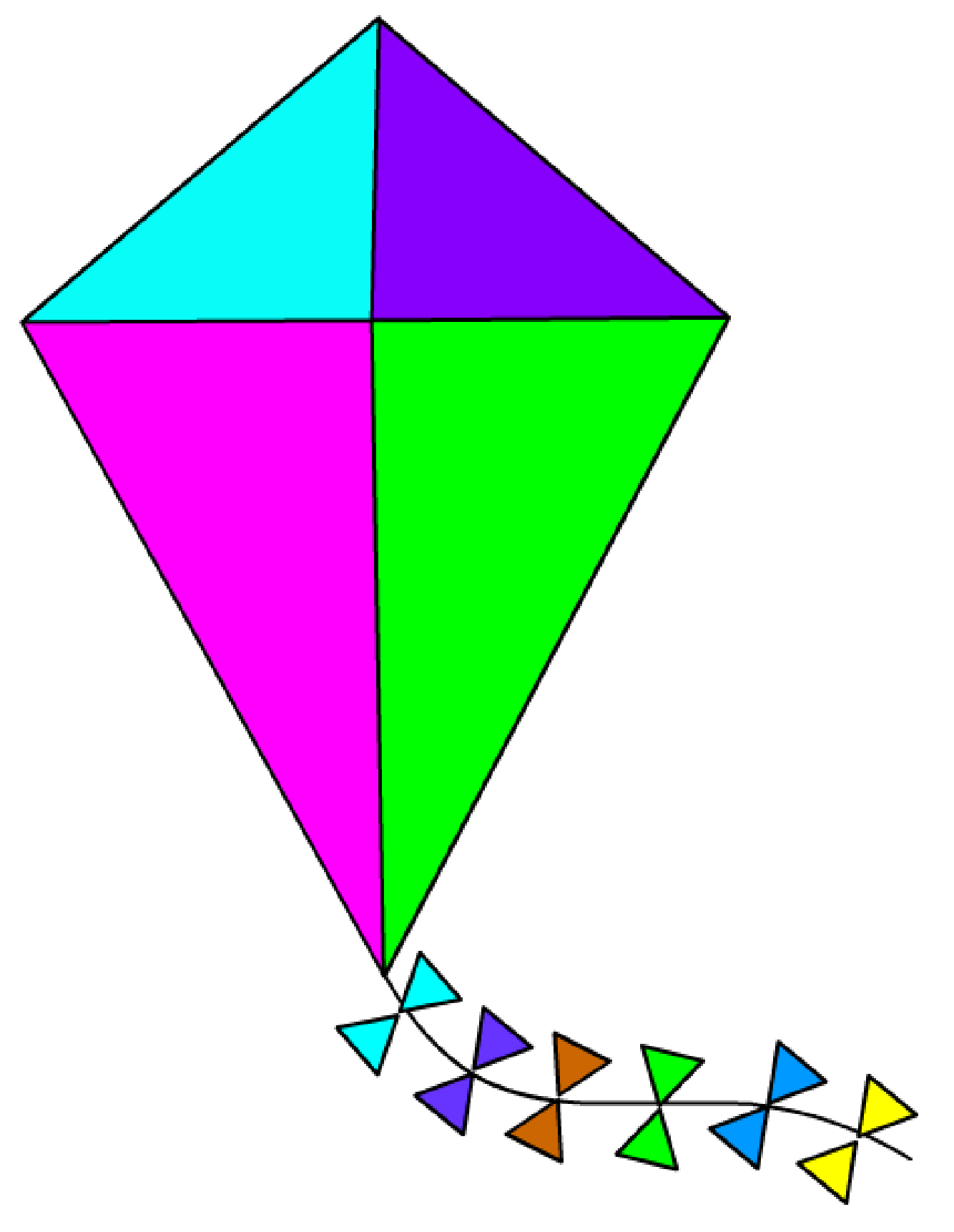Solution

No,

The first property of a regular polygon is that all the sides should be equal.

But here, the kite does not have equal sides.

Hence, it is not a regular polygon

 $$\therefore$$ Kite is not a regular polygon.
 Example 2

Calculate the sum of interior angles of an octagon.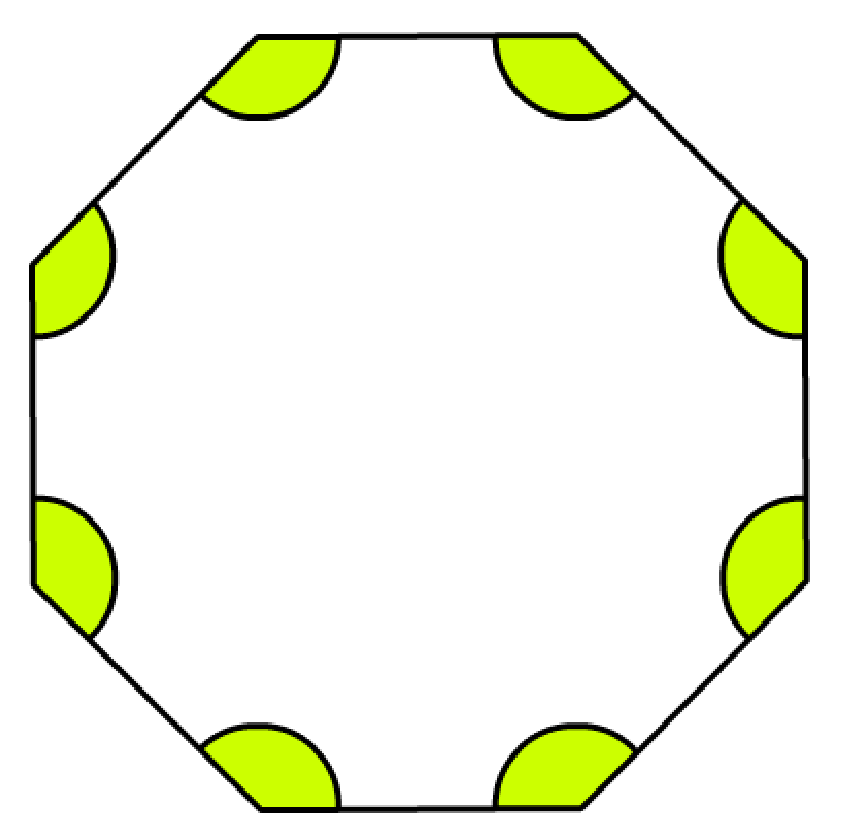Solution

We will use the formula:

Sum of Interior angles =$$\left ( n-2 \right )\times 180^{\circ}$$

Since an Octagon has 8 sides, n = 8

Sum of interior angles of the octagon =$$\left ( n-2 \right )\times 180^{\circ}$$

\begin {align}&=\left ( n-2 \right )\times 180^{\circ}\\&=\left ( 8-2 \right )\times 180^{\circ}\\&=6\times 180^{\circ}\\&=1080^{\circ} \end {align}

 $$\therefore$$1080°
 Example 3

Find the value of each interior angle of a regular pentagon.

Solution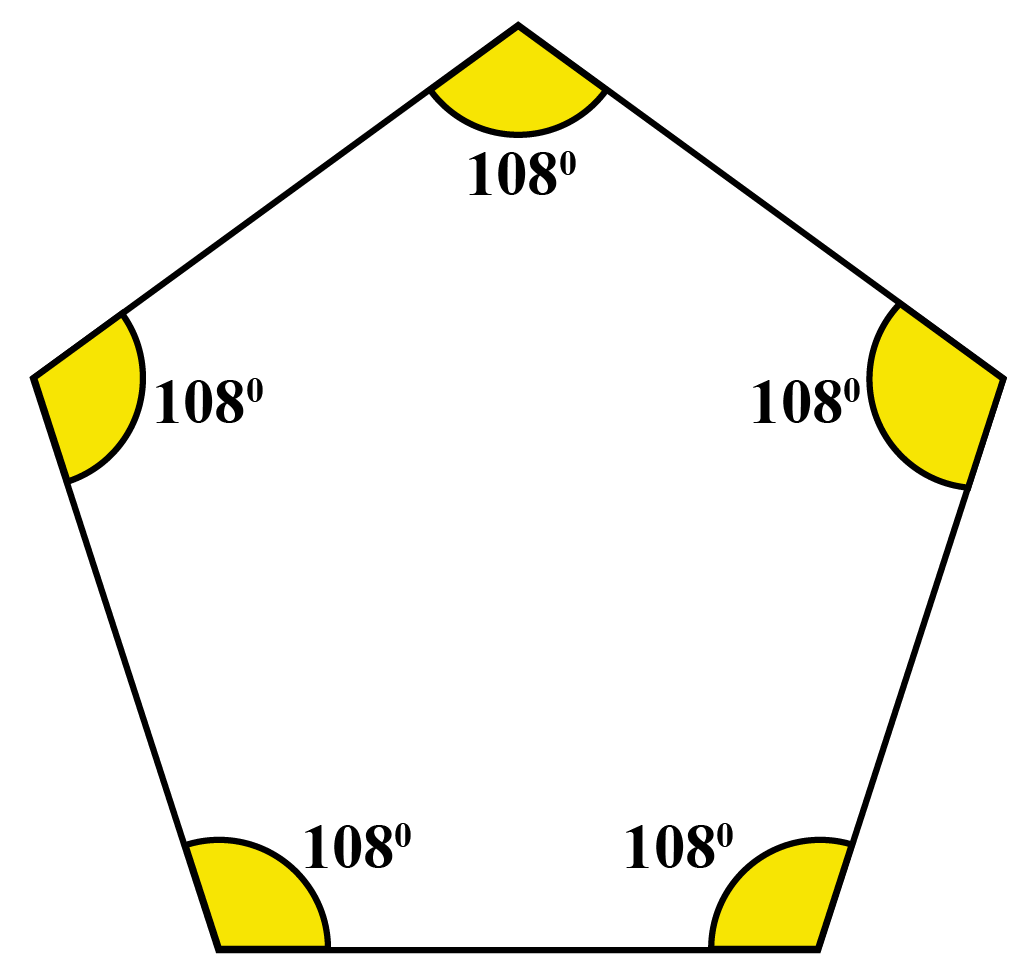We will use the formula:

Interior angle = $$\frac{\left ( n-2 \right )\times 180^{\circ}}{n}$$

Since a pentagon has 5 equal sides, n = 5 sides

Each interior angle:

\begin {align}&=\frac{\left ( n-2 \right )\times 180^{\circ}}{n}\\&=\frac{\left ( 5-2 \right )\times 180^{\circ}}{5}\\&=\frac{\left ( 3 \right )\times 180^{\circ}}{5}\\&=108^{\circ} \end {align}

 $$\therefore \text {Each interior angle is 108°}$$
 Example 4

Find the value a single exterior angle of a regular hexagon.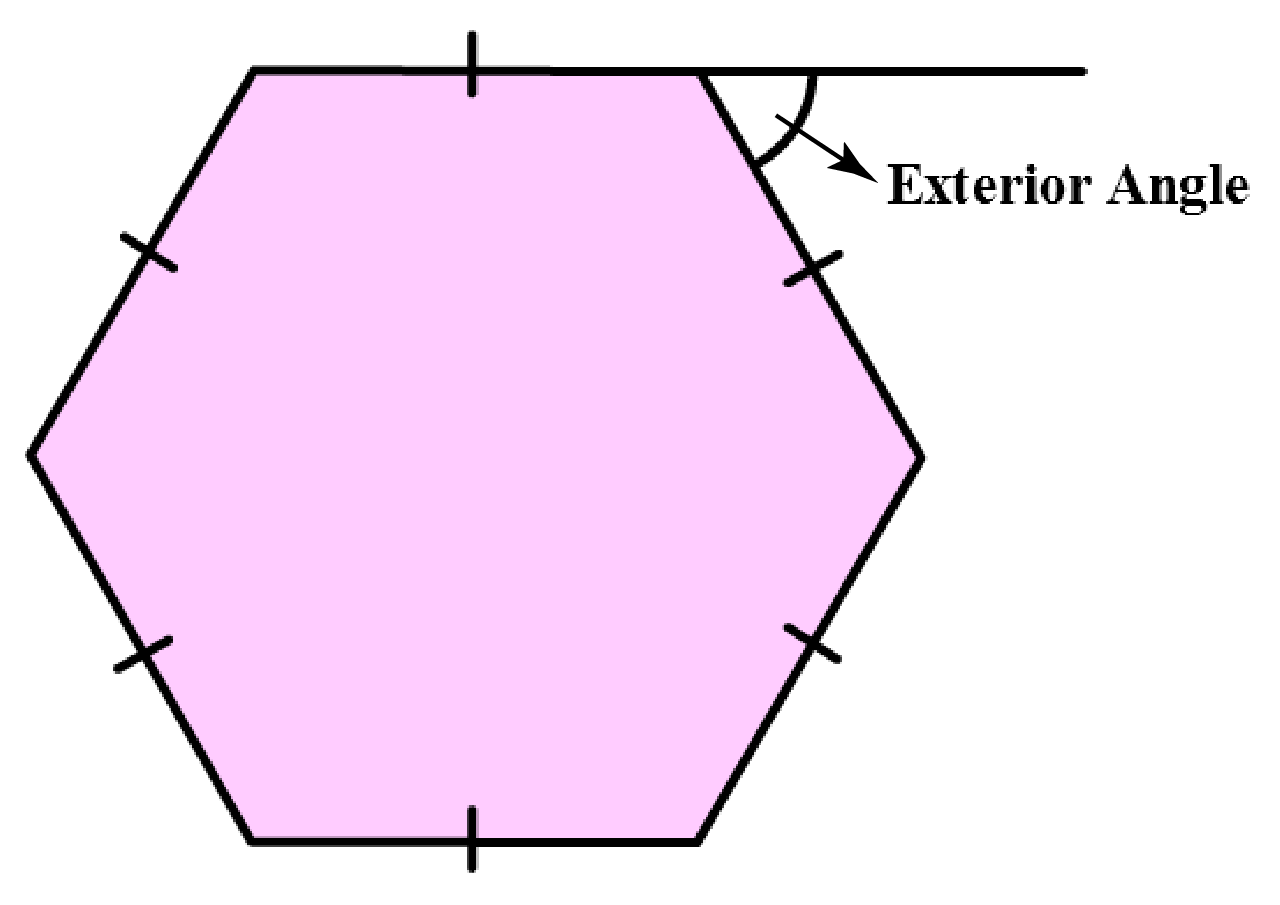Solution

We will use the formula:

Exterior angle =$$\frac{360^{\circ}}{n}$$

Since a hexagon has 6 sides, n = 6

Exterior angle:

\begin {align}&=\frac{360^{\circ}}{n}\\&=\frac{360^{\circ}}{6}\\&=60^{\circ} \end {align}

 $$\therefore \text {Exterior angle is 60°}$$
 Example 5

Find the number of sides of a regular polygon whose interior angle is 120°.

Solution

Using the formula

\begin {align}\text{Interior angle}&=\frac{\left ( n-2 \right )\times 180^{\circ}}{n}\\120&=\frac{\left ( n-2 \right )\times 180^{\circ}}{n}\\120n&=180n-360\\360&=60n\\n&=6 \end {align}

Therefore, this regular polygon has 6 sides.

 $$\therefore$$ 6 sidesChallenging Questions
1. Can Polygons be concave and regular?
2. If the sum of the interior angles of a regular polygon measures 1440°. How many sides does this polygon have?

## Interactive Questions

Here are a few activities for you to practice.

## Let's Summarize

We hope you enjoyed learning about Regular Polygons with the simulations and practice questions. Now, you will be able to easily solve problems on regular polygon examples, irregular polygons, and area of a regular polygon.

At Cuemath, our team of math experts is dedicated to making learning fun for our favourite readers, the students!

Through an interactive and engaging learning-teaching-learning approach, the teachers explore all angles of a topic.

Be it worksheets, online classes, doubt sessions, or any other form of relation, it’s the logical thinking and smart learning approach that we at Cuemath believe in.

## 1. What defines a regular polygon?

If all the sides and all the angles of a polygon are equal, it is called a regular polygon.

## 2. How many degrees are there in a regular polygon?

The degrees in a regular polygon mean the sum of interior angles of a regular polygon.

This is calculated with the help of the formula: $$\left ( n-2 \right )\times 180^{\circ}$$; where 'n' is the number of sides of the polygon.

For example, in a triangle the sum of interior angles = 180°, because the number of sides are 3

## 3. Is pentagon a regular polygon?

All pentagons may not be regular polygons. However, a pentagon can be a regular polygon if it fulfils all the following 3 properties:

All the 5 sides should be equal, all its interior angles should be equal, and all its exterior angles should be equal.

## 4. What is the area of a regular polygon?

The area of a regular polygon is the space of the interior part of the polygon.

This can be calculated with the formula : Area = \begin{align}\frac{n\times s\times a}{2}\end{align};

where n = number of sides of the polygon, s = measure of one side of the polygon, a = apothem-the distance of a perpendicular line from any side of the polygon to its center.

More Important Topics
Numbers
Algebra
Geometry
Measurement
Money
Data
Trigonometry
Calculus